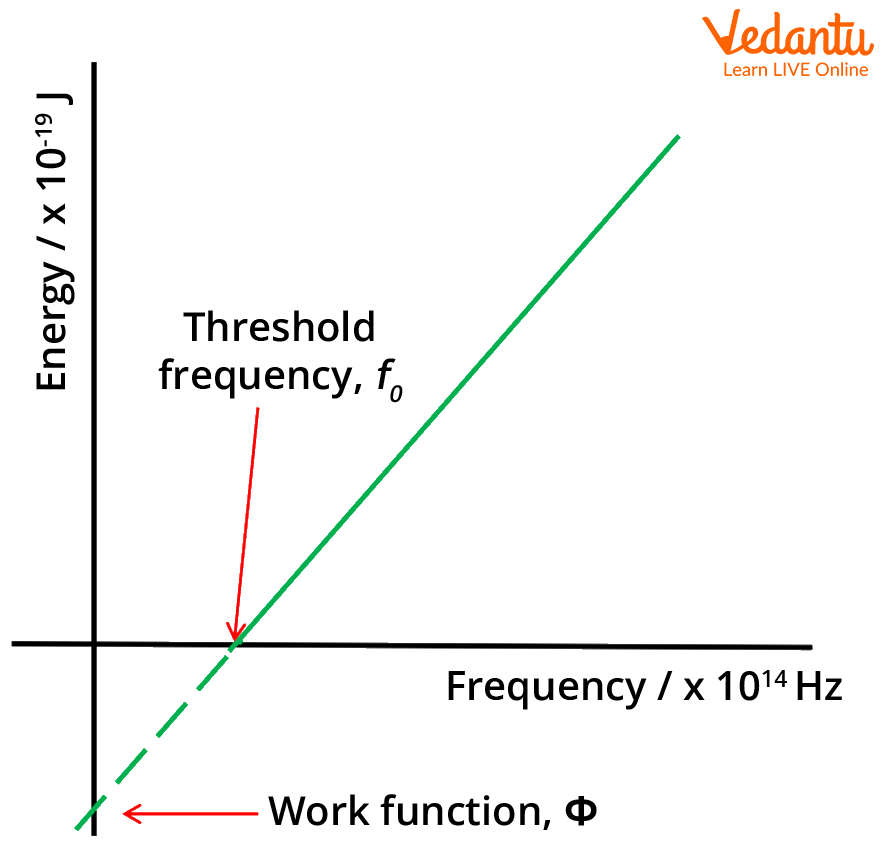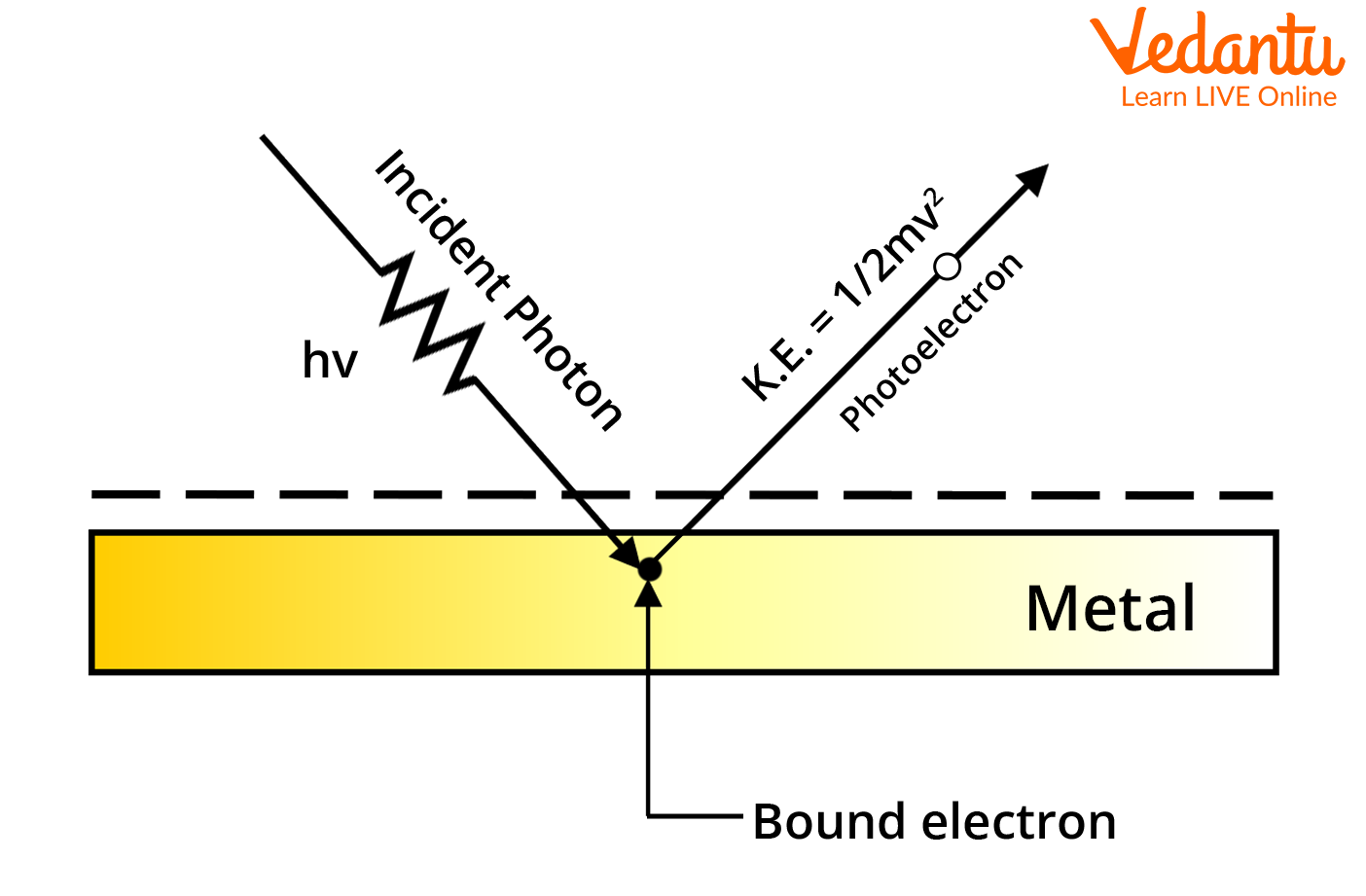Courses
Courses for Kids
Free study material
Free LIVE classes
More

# Photoelectric Effect and Stopping Potential with Work Function and Derivation for JEELIVE
Join Vedantu’s FREE Mastercalss

## What is the Photoelectric Effect?

In 1905, Albert Einstein gave his Nobel prize-winning paper on the discrete quantisation of energy in electromagnetic radiation. The core premise of this work was based on the data obtained from the photoelectric effect experiments done by Aleksandr Stoletov. To explain the photoelectric effect, they found a correlation between the intensity of light and the number of electrons emitted. According to the photoelectric effect, when an electromagnetic wave of a specific frequency (energy) is bombarded on a metal, the electrons inside the metal are ejected and become known as photoelectrons through a process known as photoemission.

## Work Function

The work function draws a barrier between emission and absorption. If the incident electromagnetic wave does not have energy equal to the work function of the material, electrons will not be ejected from the atoms of the metal. Quantised wave packets can activate emission if they have energies greater than the work function of the metal.

In solid-state physics, the work function is the minimum thermodynamic work needed to remove an electron from a solid to a point in the vacuum immediately outside the solid surface. Work function is a property of a material, which is defined as the minimum quantity of energy required to remove an electron to infinity from the surface of a given solid.Energy  versus frequency graph

## What is Stopping Potential?

Stopping potential, or cut-off potential, is defined as the required potential for stopping the removal of an electron from a metal surface when the incident light energy is greater than the work potential of the metal on which the incident light is focused. The work function and stopping potential tend to mean the same thing in terms of the photoelectric effect.

## Derivation of Photoelectric Effect and Stopping Potential Formula

To derive Einstein’s photoelectric equation formula for stopping potential, we need to identify the required variables and parameters first. As we know

$c=\nu \lambda$

where c is the speed of light, 𝛎 is the frequency of the electromagnetic wave, and $\lambda$ is the measure of the wavelength of the electromagnetic wave.

$\nu=\dfrac{c}{\lambda}$

$\Rightarrow E=h \nu$……..(1)

Here, E is the energy of the electromagnetic wave.

Therefore, we can use the known parameters to calculate the kinetic energy of the photoelectrons.

$K E_{\max }=\dfrac{h c}{\lambda}-\phi$……..(2)

The stopping potential $\phi$ can be given as

$\phi=\dfrac{h c}{\lambda}-K E_{\max }$Process of Photoemission

Now the kinetic energy can be written as

$K E_{\max }=\dfrac{1}{2} m v_{\max}^{2}$

Also, the energy of a photo equal to the work function can be written as

\begin{align} &\phi=\dfrac{h c}{\lambda_{0}} \\ &\phi=h \nu_{0} \end{align}

So, the equation for the stopping potential can be written as

\begin{align} &h \nu_{0}=\dfrac{h c}{\lambda}-\dfrac{1}{2} m v_{\max }^{2} \\ &h \nu_{0}=h \nu-\dfrac{1}{2} m v_{\max }^{2} \end{align}

We can also write

\begin{align} &\dfrac{1}{2} m v_{\max }^{2}=h \nu-h v_{0} \\ &\dfrac{1}{2} m v_{\max }^{2}=h\left(\nu-\nu_{0}\right) \end{align}

The photoelectric effect can be derived from the concept of Planck's quantum theory. Photons travel in discrete energy packets, where the energy of one photon is given by the equation (1). $\nu$ is the frequency of the incident photon. ‘h’ is the Planck’s constant whose value is $h=6.6260715 \times 10^{-34} \mathrm{~J} . \mathrm{s}$ the units are Joule-Second.

To summarise the variables used in the derivation of the photoelectric equation, we have

• h, which is the Planck constant.

• $\nu$, which is the frequency of the radiation in question.

• $\lambda$, which is the wavelength of the radiation.

• c, which is the speed of light in a vacuum.

• E, which is the energy of the photon.

There is a single interaction that sums up the photoelectric effect, the incidence of a photon on the metal surface, and its interaction with the electron bound to the surface of the metal within the interatomic interactions.

## Dependence of Photoelectric Effect on Different Parameters

1. For a light of any given frequency, the photoelectric current is directly proportional to the intensity of light.

2. If the frequency of light is no greater than the threshold frequency, the emission of photoelectrons will be stopped, no matter how intense the light rays are.

3. The maximum kinetic energy of the photoelectrons emitted is independent of the intensity of light. As the frequency of light increases over the threshold frequency, the kinetic energy of the electrons also increases.

4. The process of photoemission is instantaneous, as it depends on the quanta of energy the radiation brings with it.

## Conclusion

The phenomenon of the photoelectric effect was discovered by the works of Heinrich Hertz, Albert Einstein, Max Planck, and others during the late 19th to early 20th century. The photoelectric effect can be defined as a phenomenon during which electrons are emitted from the surface of the metal when the light of a suitable wavelength is incident on it. These electrons are termed photoelectrons.

This emission occurs due to the electrons absorbing energy from the electromagnetic radiation and using this agitation to break free from the intra-atomic forces of attraction in the metal. This spontaneous emission of electrons is called photoemission. The current generated due to this emission is referred to as photocurrent. This phenomenon converts light energy into electrical energy.

Last updated date: 20th Sep 2023
Total views: 131.4k
Views today: 2.31k

## FAQs on Photoelectric Effect and Stopping Potential with Work Function and Derivation for JEE

1. How many questions are asked about the photoelectric effect in JEE?

Around 1-2 questions would be asked on this topic for the exam which makes around 4-8 marks in the total of the exam. Preparing this concept will help you gain these easy marks in the total. Preparing previous years’ questions will help you to understand how the questions are framed on related concepts and will give you an idea of how to tackle such problems with ease. Try to search for unusual problems to get insight into the relevance of the photoelectric effect in JEE.

2. What is the physical significance of the photoelectric effect?

The photoelectric effect proves the behaviour of light as a particle-type nature. This is in direct contrast with the theory of the wave nature of light, which is shown in experiments related to interference, diffraction, etc. For studying both the natures of light as a wave and as a particle, this phenomenon of the photoelectric effect is very crucial. It’s true that the energy of the radiation is uniformly distributed along the wavefront of the electromagnetic wave, however, Planck and Einstein proved that the light energy is quantised in the form of wave packets known as quanta.